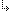- 2.2.9.6. -2. TMT Pascal Language Description2.2. Pascal Language Structure2.2.9. Statements2.2.9.6. If Statement

## 2.2.9.6. If Statement

The If statement conditionally executes one of two statements based on the value of an expression. If statements take the following form:
`if expression then statement [else statement]`
where expression evaluates to a Boolean value. If expression results in True then the statement following the reserved word then is executed. Control is then transferred to the first statement outside the if statement.

If expression evaluates to False and an else clause is specified then the statement following else is executed. If no else clause exists and expression is False then the if statement is passed over. The following is an example of an if statement.
```if Flag then
WriteLn('Expression is True')
else
WriteLn('Expression is False');```
The end of an if statement is indicated by a semicolon (;). In the above example, if Flag is a constant then TMT Pascal optimizes code generation and automatically eliminates code that is never executed.

- 2.2.9.6. -